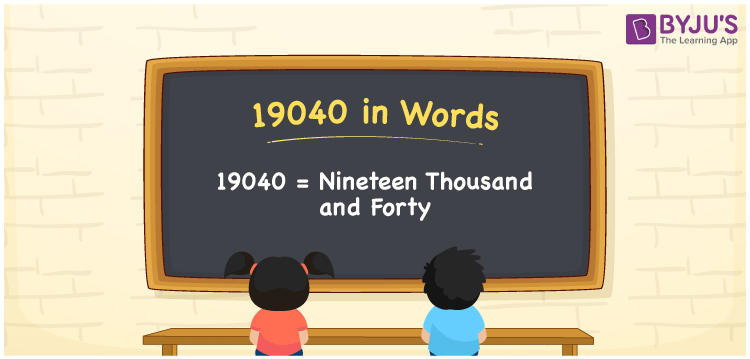# 19040 in Words

We can express the number 19040 in words as Nineteen Thousand and Forty. For example, Rohan spent Rs. 19040 for his birthday party yesterday, then you can express the same sentence in words as “Rohan spent Rupees Nineteen Thousand and Forty for his birthday party yesterday”. We know that the number 19040 is a cardinal number or counting number as it depicts an exact value. Place value chart is very helpful to write the numbers into words accurately. This article provides a complete explanation about how to write the number 19040 in words.

 19040 in Words Nineteen Thousand and Forty Nineteen Thousand and Forty in numerical form 19040

## 19040 in English Words

Generally, in Mathematics, the letters of the English alphabet are used to write the numbers in words. Hence, we can write and spell the number 19040 in English as Nineteen Thousand and Forty.## How to Write 19040 in Words?

We use a place chart to represent the numerical name for the given number. The following table shows the place value chart for 19040.

 Ten-Thousands Thousands Hundreds Tens Ones 1 9 0 4 0

Therefore, we can write the expanded form as:

1 x Ten Thousand + 9 x Thousand + 0 x Hundred + 4 x Ten + 0 x One

= 1 x 10000 + 9 x 1000 + 0 x 100 + 4 x 10 + 0 x 1

= 10000 + 9000 + 0 + 40 + 0

= 10000 + 9000 + 40

= 19040

= Nineteen Thousand and Forty

Hence, 19040 in words is written as Nineteen Thousand and Forty

Interesting way of writing 19040 in words

1 = One

19 = Nineteen

190 = One Hundred and Ninety

1904 = One Thousand Nine Hundred Four

19040 = Nineteen Thousand and Forty

Thus, the word form of the number 19040 is Nineteen Thousand and Forty

19040 is a natural number that precedes 19041 and succeeds 19039

• 19040 in words – Nineteen Thousand and Forty
• Is 19040 an odd number? – No
• Is 19040 an even number? – Yes
• Is 19040 a perfect square number? – No
• Is 19040 a perfect cube number? – No
• Is 19040 a prime number? – No
• Is 19040 a composite number? – Yes

## Frequently Asked Questions on 19040 in Words

Q1

### How to write 19040 in words?

We write 19040 in words as Nineteen Thousand and Forty.
Q2

### Simplify 18000 + 1040, and express in words.

Simplifying 18000 + 1040, we get 19040. Hence, the number 19040 in words is written as Nineteen Thousand and Forty.
Q3

### How to write Nineteen Thousand and Forty in numbers?

Nineteen Thousand and Forty in numbers is 19040.